# 10-327/Classnotes for Monday December 6

• No, the compactness of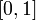$[0,1]$ is not used. As we said in class, if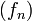$(f_n)$ is Cauchy in the uniform metric, then for any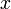$x$, the sequence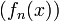$(f_n(x))$ is Cauchy in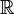${\mathbb R}$, so it has a limit. Call that limit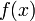$f(x)$; it is not hard to show that$f$ is continuous and that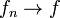$f_n\to f$. Theorem 43.6 in Munkres is a slight generalization of this. Drorbn 07:12, 20 December 2010 (EST)